# Class 11 RD Sharma Solutions – Chapter 6 Graphs of Trigonometric Functions – Exercise 6.1

### (i) y = 2 sin 2x

Solution:

To obtain this graph y = 2 sin 2x,

First we draw the graph of y = sin x and then divide the x-coordinates of the points where it crosses x-axis by 2.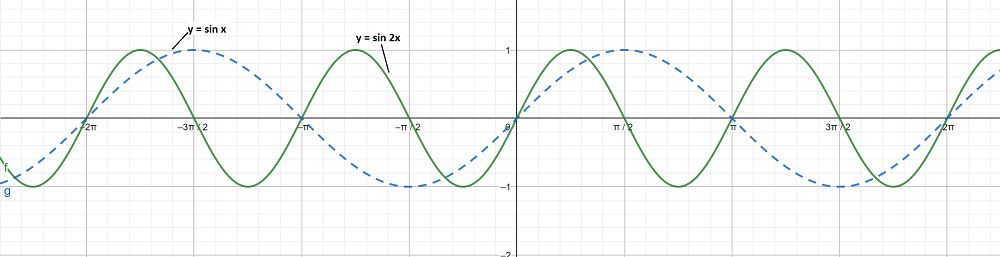The maximum and minimum values of y are 2 and -2 respectively.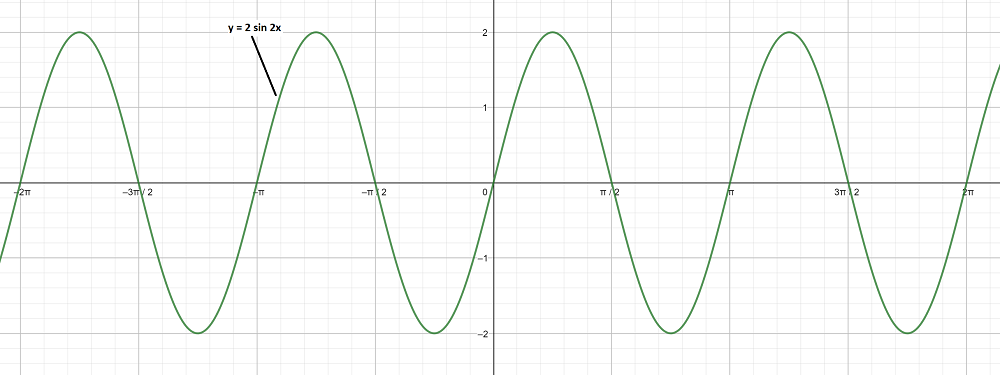### (ii) y = 3 sin x

Solution:

To obtain this graph y = 3 sin x,

First we draw the graph of y = sin x.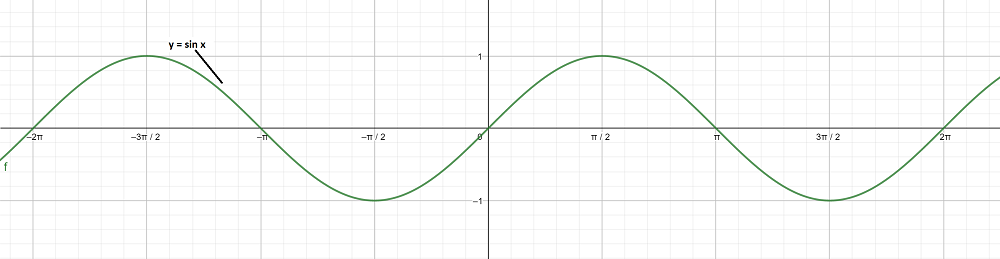The maximum and minimum values of y are 3 and -3 respectively.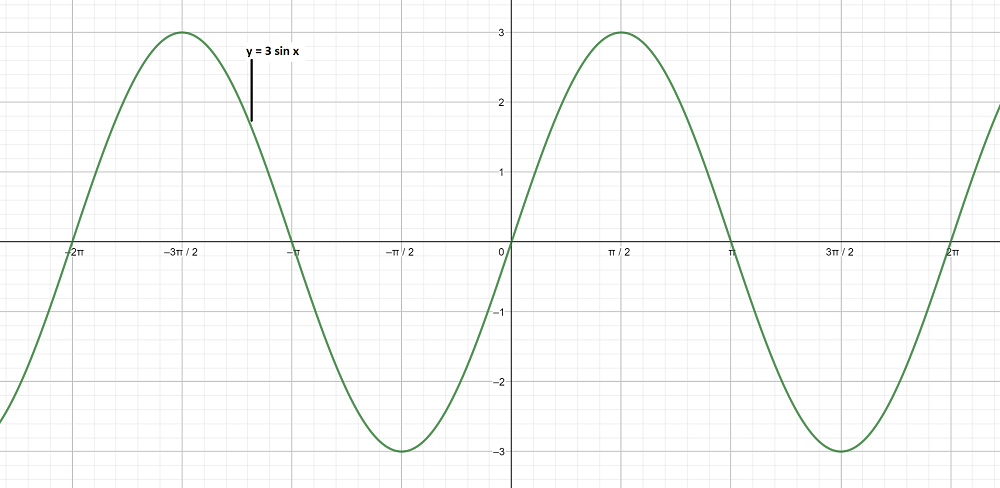### (iii)Solution:

To obtain this graph y-0 =,

On shifting the origin at (π/4, 0), we get

X =and Y = y – 0

On substituting these values, we get

Y = 2 sin X

First we draw the graph of Y = 2 sin X and shift it by π/4 to the right.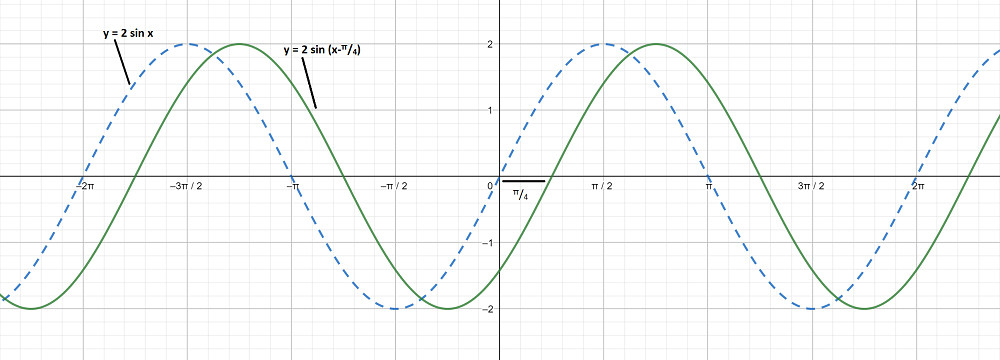### (iv) y = 2 sin (2x – 1)

Solution:

To obtain this graph y – 0 = 2 sin,

On shifting the origin at (1/2, 0), we get

X =and Y = y – 0

On substituting these values, we get

Y = 2 sin 2X

First we draw the graph of Y = 2 sin 2X and shift it by 1/2 to the right.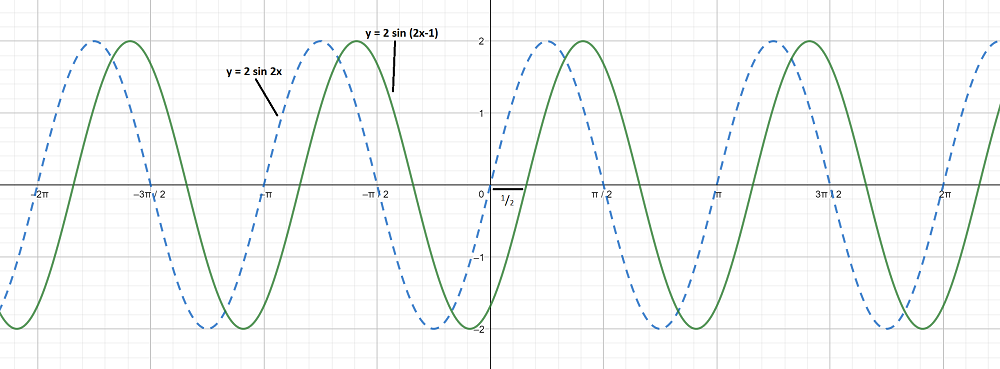### (v) y = 3 sin (3x + 1)

Solution:

To obtain this graph y – 0 = 3 sin 3,

On shifting the origin at(-1/3, 0), we get

X =and Y = y – 0

On substituting these values, we get

Y = 3 sin 3X

First we draw the graph of Y = 3 sin 3X and shift it by 1/3 to the left.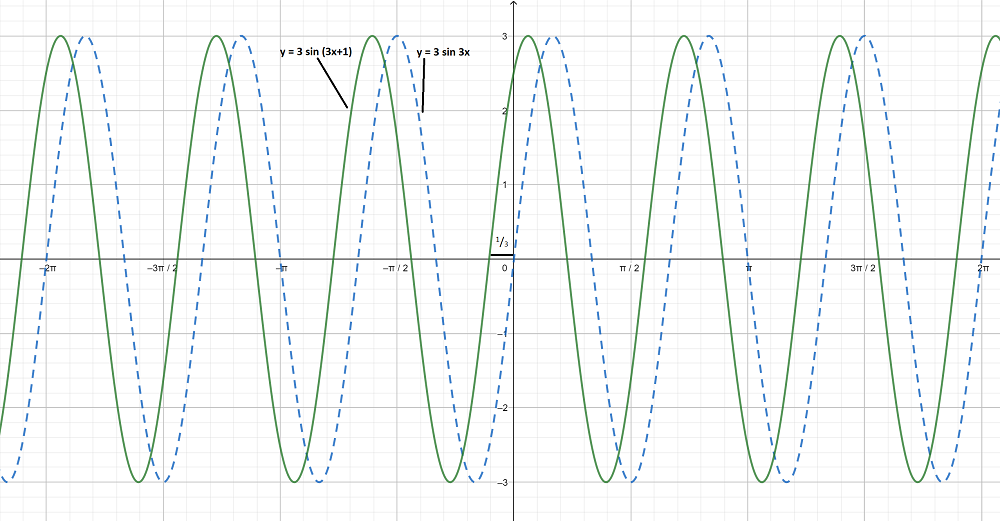### (vi)To obtain this graph y-0 =On shifting the origin at (π/8, 0), we get

X =and Y = y-0

On substituting these values, we get

Y = 3 sin 2X

First we draw the graph of Y = 3 sin 2X and shift it by π/8 to the right.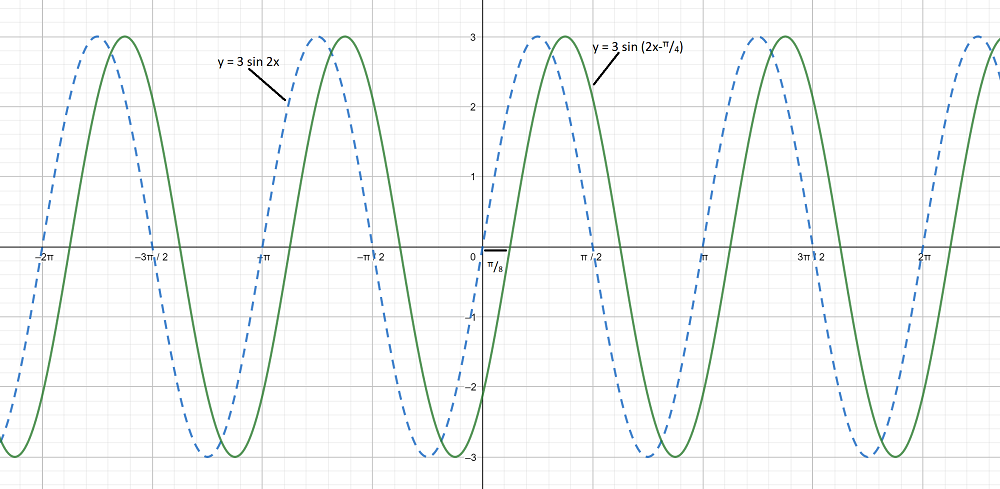### (i) y = sin x,Graph 1:

y = sin x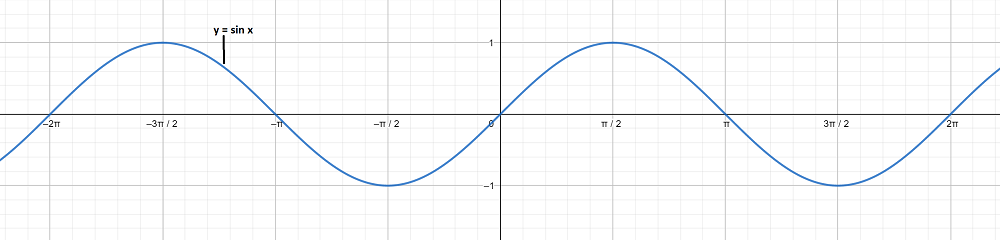Graph 2:

To obtain this graph y-0 =On shifting the origin at (-π/4, 0), we get

X =and Y = y – 0

On substituting these values, we get

Y = sin X

First we draw the graph of Y = sin X and shift it by π/4 to the left.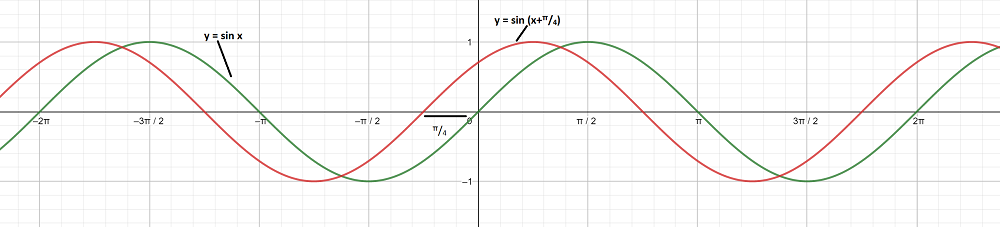The graph y = sin x andare on different axes are as follows: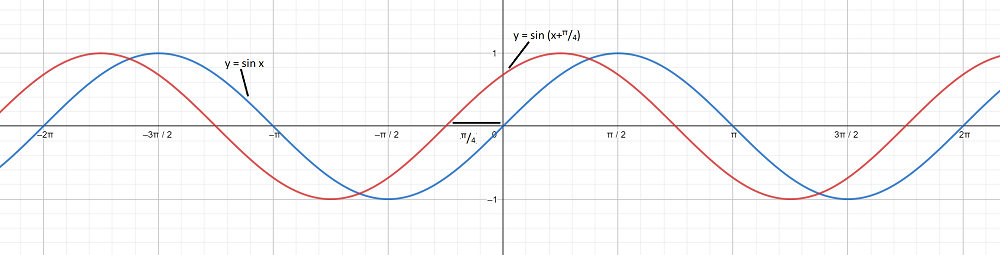### (ii) y = sin x, y = sin 3x

Graph 1:

y = sin x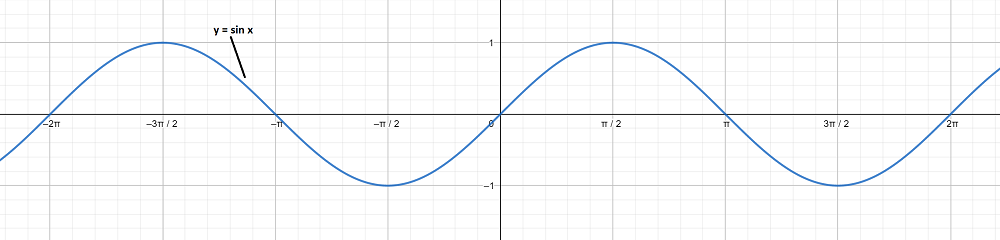Graph 2:

To obtain this graph y = sin 3x,

First we draw the graph of y = sin x and then divide the x-coordinates of the points where it crosses x-axis by 3.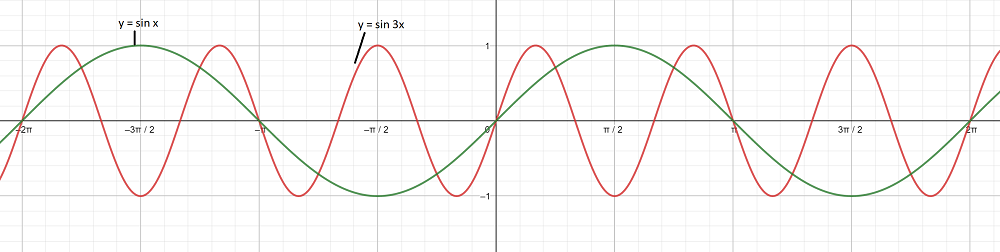The graph y = sin x and y = sin 3x are on different axes are as follows: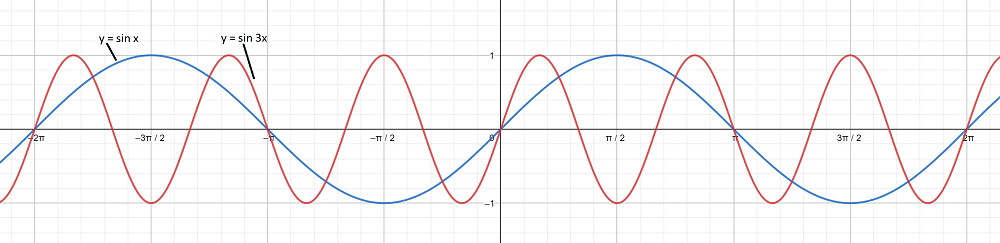Whether you're preparing for your first job interview or aiming to upskill in this ever-evolving tech landscape, GeeksforGeeks Courses are your key to success. We provide top-quality content at affordable prices, all geared towards accelerating your growth in a time-bound manner. Join the millions we've already empowered, and we're here to do the same for you. Don't miss out - check it out now!

Related Tutorials